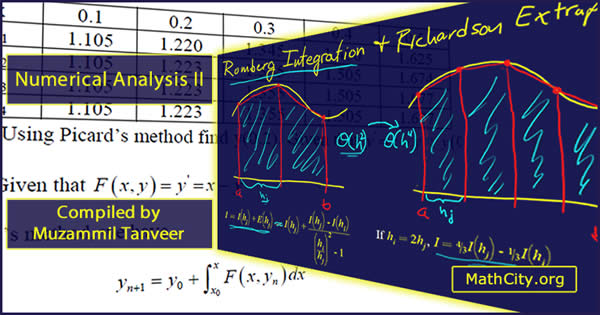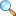Numerical Analysis IIThese notes are provided and composed by Mr. Muzammil Tanveer. We are really very thankful to him for providing these notes and appreciates his effort to publish these notes on MathCity.org.

 Name Numerical Analysis II Muzammil Tanveer 128 pages PDF 4.66 mB

Summary & contents

• Operator
• Difference Equation
• Homogeneous Linear Difference Equation
• Non-Homogeneous Linear Difference Equation
• Non-Homogeneous Difference Equation
• Formation of Difference Equation
• Numerical Integration
• Trapezoidal Rule
• Simpson’s 1/3 Rule
• Simpson’s 3/8 rule
• Bool’s Rule
• Weddle’s Rule
• Numerical solution of ODE’s (ordinary differential equations) or Initial value problem
• Picard’s Method
• Euler’s Method
• Semi-Implicit Euler’s Method
• Implicit Euler’s Method
• Modified Euler’s method (Trapezoid method)

Please click on View Online to see inside the PDF.

• Download PDF (4.66 MiB, 15727 downloads) |View Online